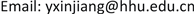1. 引言

{ d x d t = f ( x ) − p ( x , y ) x d y d t = − c y + q ( x , y ) y (1.1)

{ d x d t = a x 2 ( k − x ) − b x y + h ( x ) d y d t = − c y + ( β x − α y ) y (1.2)

{ d x d t = a x 2 ( k − x ) − b x y + h x 2 d y d t = − c y + ( β x − α y ) y (1.3)

2. 系统平衡点分析

{ d x d t = x ( − a 2 x 2 + a 1 x − a 0 y ) = R ( x , y ) d y d t = y ( x − 1 − y ) = S ( x , y ) (2.1)

O ( 0 , 0 ) , A ( a 1 a 2 , 0 ) , B ( x B , y B )

1) 对平衡点O，其系数矩阵 J ( O ) = ( 0 0 0 − 1 ) ，由此可知两个方程的特征根分别为0，−1，该点为系统的一个鞍点。

2) 对平衡点A，其特征根为 λ 1 = − a 1 2 a 2 , λ 2 = a 1 a 2 − 1 ，显然 − a 1 2 a 2 < 0 ，所以，当 a 1 a 2 − 1 > 0 即 a 1 > a 2 时，A为系统鞍点；当 a 1 a 2 − 1 < 0 即 a 1 < a 2 时，A为系统的稳定结点，且所有特征值均具有负实部，所以结点A是渐近稳定的 。

3) 对于平衡点B，可以分成如下三种情况进行讨论：

a) 当 a 1 > a 0 ， a 1 − a 0 > a 2 时，易知 ( a 1 − a 0 ) 2 + 4 a 0 a 2 > a 1 − a 0 ，所以有 x B > a 1 − a 0 a 2 > 1 ， y B > 0 ，系统存在正平衡点。

b) 当 a 1 = a 0 ， a 0 > a 2 时， x B = a 0 a 2 a 2 = a 0 a 2 > 1 ， y B > 0 ，系统存在正平衡点。

c) 当 a 1 < a 0 时，若想要系统存在正平衡点，则需让 x B > 1 ，整理得 a 2 < a 1 时，所以得 a 2 < a 1 < a 0 时系统存在正平衡点。

d e t ( J ( B ) ) = ( − 3 a 2 x B 2 + 2 a 1 x B − a 0 y B ) ( x B − 1 − 2 y B ) + a 0 x B y B = x B y B ( a 1 − a 0 ) 2 + 4 a 0 a 2 > 0

T = − 3 a 2 x B 2 + 2 a 1 x B − a 0 y B + x B − 1 − 2 y B = ( 2 a 0 − a 1 − 1 ) [ ( a 1 − a 0 ) + ( a 1 − a 0 ) 2 + 4 a 0 a 2 ] 2 a 2 − 2 a 0 + 1

3. 极限环的存在性

1) 当 x ( 0 ) < a 1 a 2 时， ∀ t ≥ 0 ， x ( t ) ≤ a 1 a 2 。若存在 t 1 < 0 ，使得 x ( t 1 ) = a 1 a 2 且 d x d t | t = t 1 ≥ 0 ，

2) 若 N ≥ a 1 a 2 ，则 d x d t | x = N = N ( a 1 N − a 2 N 2 − a 0 y ) < 0 ，所以 x = N ( N ≥ a 1 a 2 ) 是系统的无切线且轨线是穿向 x = N 的左边，因此 ∀ t ≥ 0 ， x ( t ) ≤ x ( 0 ) 。

d C d t | C = 0 = d y d t + 1 a 0 d x d t | y + x a 0 − c = 0 = − a 2 a 0 x 3 + a 1 a 0 x 2 + x a 0 − y 2 − c

x B − x 0 = ( a 1 − a 0 ) + ( a 1 − a 0 ) 2 + 4 a 0 a 2 2 a 2 − a 1 a 2 = − ( a 1 + a 0 ) + ( a 1 − a 0 ) 2 + 4 a 0 a 2 2 a 2

∂ ( B R ) ∂ x + ∂ ( B S ) ∂ y = − a 2 y + a 0 − 1 x 2 < 0

4. 数值模拟

5. 系统的生态意义